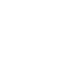MDX

# Using Linear Regression to Calculate Growth

A few blog entries back I showed the MDX I used to calculate a seasonally-adjusted total in my chalk talk at the BI conference. This is useful but if we’re looking for a calculation that we can use for the Trend property of a KPI it’s not the whole story – we still need to find a way of expressing how much a value is growing or shrinking over time. Although previous period growth calculations are a lot more useful with seasonally-adjusted values, we can use simple linear regression (and it has to be simple because, as I said, I’m no statistician) to do a better job.

The starting point for understanding how to use linear regression in MDX is (surprise, surprise) Mosha’s blog entry on the subject:

http://sqljunkies.com/WebLog/mosha/archive/2004/12/21/5689.aspx

However, the function that’s going to be most useful here is the linregslope function. If we’re looking at the values in our time series and trying to find a line of best fit for those values with the equation y=ax+b, linregslope returns the value of a in that equation, ie the gradient – when the value of x increases by 1, y increases by the value of a. Here’s an example of how to use it:

linregslope(
lastperiods(3, [Date].[Calendar].currentmember) as last3
, [Measures].[Internet Sales Amount]
,rank([Date].[Calendar].currentmember, last3)
)

select {[Measures].[Internet Sales Amount], measures.gradient} on 0,
[Date].[Calendar].[Month].members on 1
1.Steve says: